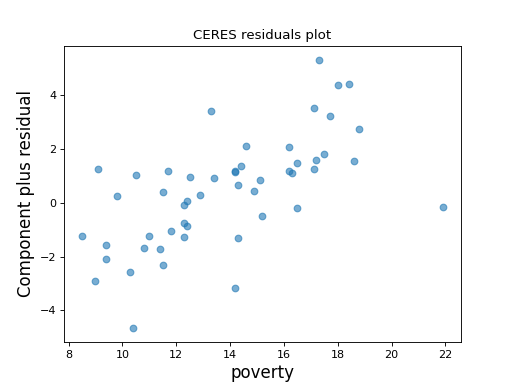# statsmodels.graphics.regressionplots.plot_ceres_residuals¶

statsmodels.graphics.regressionplots.plot_ceres_residuals(results, focus_exog, frac=0.66, cond_means=None, ax=None)[source]

Produces a CERES (Conditional Expectation Partial Residuals) plot for a fitted regression model.

Parameters
results: object

Results for a fitted regression modelfocus_exog : integer or string The column index of results.model.exog, or the variable name, indicating the variable whose role in the regression is to be assessed.

fracfloat

Lowess tuning parameter for the adjusted model used in the CERES analysis. Not used if cond_means is provided.

cond_meansarray-like, optional

If provided, the columns of this array span the space of the conditional means E[exog | focus exog], where exog ranges over some or all of the columns of exog (other than the focus exog).

axmatplotlib.Axes instance, optional

The axes on which to draw the plot. If not provided, a new axes instance is created.

Returns
figmatplotlib.Figure instance

The figure on which the partial residual plot is drawn.

Notes

cond_means is intended to capture the behavior of E[x1 | x2], where x2 is the focus exog and x1 are all the other exog variables. If all the conditional mean relationships are linear, it is sufficient to set cond_means equal to the focus exog. Alternatively, cond_means may consist of one or more columns containing functional transformations of the focus exog (e.g. x2^2) that are thought to capture E[x1 | x2].

If nothing is known or suspected about the form of E[x1 | x2], set cond_means to None, and it will be estimated by smoothing each non-focus exog against the focus exog. The values of frac control these lowess smooths.

If cond_means contains only the focus exog, the results are equivalent to a partial residual plot.

If the focus variable is believed to be independent of the other exog variables, cond_means can be set to an (empty) nx0 array.

References

RD Cook and R Croos-Dabrera (1998). Partial residual plots in generalized linear models. Journal of the American Statistical Association, 93:442.

RD Cook (1993). Partial residual plots. Technometrics 35:4.

Examples

Using a model built from the the state crime dataset, make a CERES plot with the rate of Poverty as the focus variable.

>>> import statsmodels.api as sm
>>> import matplotlib.pyplot as plt
>>> import statsmodels.formula.api as smf
>>> from statsmodels.graphics.regressionplots import plot_ceres_residuals

>>> crime_data = sm.datasets.statecrime.load_pandas()
>>> results = smf.ols('murder ~ hs_grad + urban + poverty + single',
...                   data=crime_data.data).fit()
>>> plot_ceres_residuals(results, 'poverty')
>>> plt.show()